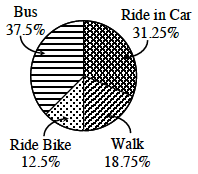### Home > ACC7 > Chapter 7 Unit 8 > Lesson CC3: 7.1.1 > Problem7-8

7-8.

A group of classmates surveyed how other students travel to school. The results of the survey are shown in the table at right. Make a circle graph showing the results of the survey using percents.

Mode of Transportation

Number of Students

Bus

$90$

Ride Bike

$30$

Ride in Car

$75$

Walk

$45$

Find the students using each mode of transportation as a percentage of all the students.

Each category of data is then represented by a portion of the circle. The measure of the angle of each sector portion is directly proportional to the corresponding percentage.

Consider the bike riders.

$\frac{30}{240}=0.125$ of the student population ride bikes to school.

Proportionately as an angle, this would be $12.5\%$ of$360°$ (a whole circle). This equates to $0.125(360°)=45°$.